# 7.5 Catalogue statistics and plots

## 7.5.1 Introduction

Author(s): Raúl Borrachero and Frédéric Arenou

The data fields of the main table of the Gaia Catalogue and a short description of these fields are indicated Table 7.4. A more extensive description of these fields is available where all other tables concerning variability and crossmatch are also described.

The TGAS subset has the same content plus two more fields, Hipparcos and Tycho-2_id which are respectively the Hipparcos identifier and the Tycho-2 identifier.

A summary of statistics and plots by Gaia analysis Tool (GAT) on these fields for the 1 142 679 769 sources is shown Table 7.5 and following pages.

## 7.5.2 Sky Density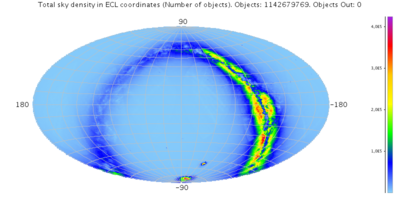Figure 7.11: HEALPix map of total density count in ecliptic coordinates (larger), logarithmic counts (larger), and in galactic coordinates (larger), logarithmic counts (larger).

## 7.5.3 Magnitude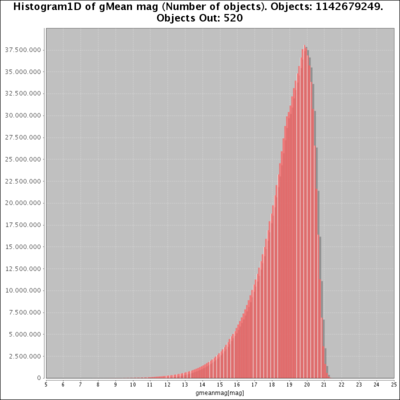Figure 7.12: Histogram of the mean G magnitude (larger), σG (larger), mean G flux (larger).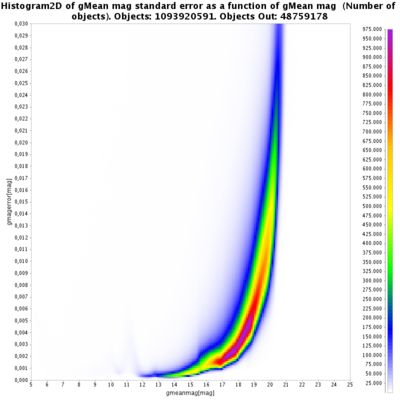Figure 7.13: Histogram 2D of the mean G magnitude as a function of the σG magnitude (larger), and as a function of the number of observations (larger).Figure 7.14: HEALPix map in ecliptic coordinates of median values of mean G magnitude (larger), median values of σG (larger), standard deviation of mean G magnitude (larger) and standard deviation of σG (larger).

## 7.5.4 PositionsFigure 7.15: Histogram of α (larger), and σα⁣* (larger).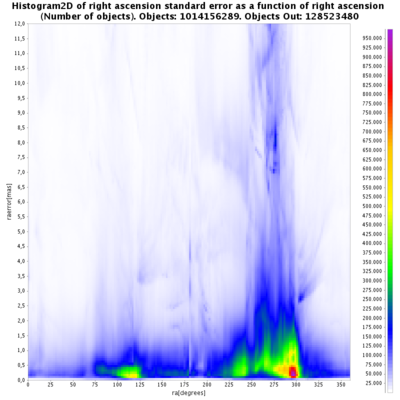Figure 7.16: Histogram 2D of σα⁣* as a function of α (larger), as a function of the G magnitude (larger), and as a function of the number of observations (larger).Figure 7.17: HEALPix map in ecliptic coordinates of σα⁣*, median value (larger), and standard deviation (larger).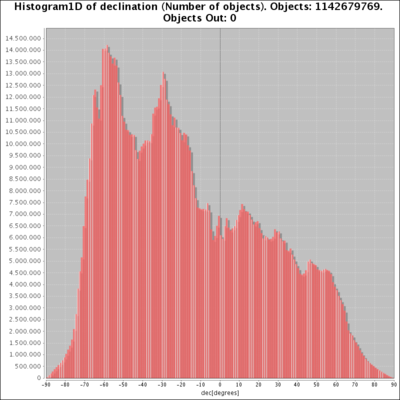Figure 7.18: Histogram of δ (larger), and of σδ (larger).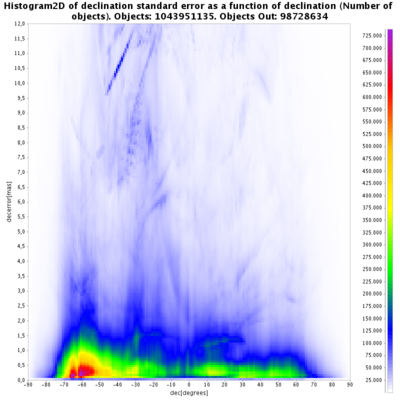Figure 7.19: Histogram 2D of σδ as a function of δ (larger), as a function of the G magnitude (larger), and as a function of the number of observations (larger).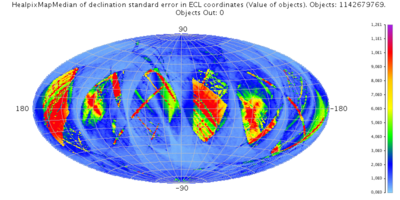Figure 7.20: HEALPix map in ecliptic coordinates of σδ: median values (larger), and standard deviation (larger).

## 7.5.5 Parallaxes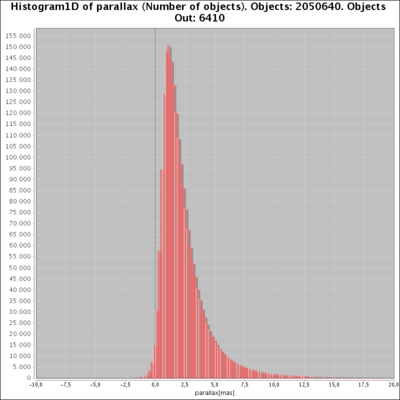Figure 7.21: Histogram of ϖ (larger), and σϖ (larger).Figure 7.22: Histogram 2D of σϖ as a function of ϖ (larger), as a function of G magnitude (larger), and as a function of the number of observations along-scan (larger).Figure 7.23: HEALPix map in ecliptic coordinates of median values of ϖ (larger), median values of σϖ (larger), and standard deviation of σϖ (larger).

## 7.5.6 Proper motions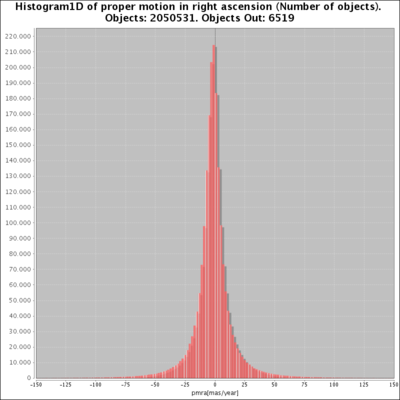Figure 7.24: Histogram of μα⁣* (larger), of σμα⁣*: linear scale (larger), and log scale (larger).Figure 7.25: Histogram 2D of σμα⁣* as a function of μα⁣* (larger), as a function of G magnitude (larger), and as a function of the number of observations along-scan (larger).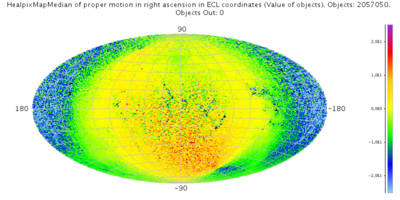Figure 7.26: HEALPix map in ecliptic coordinates of the median value of μα⁣* (larger), median value of σμα⁣* (larger), standard deviation of σμα⁣* (larger).Figure 7.27: Histogram of μδ (larger), of σμδ: linear scale (larger), and log scale (larger).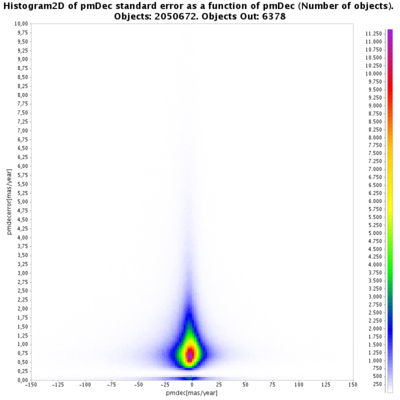Figure 7.28: Histogram 2D of σμδ as a function of μδ (larger), as a function of G magnitude (larger), and as a function of the number of observations (larger).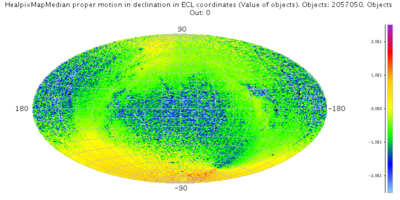Figure 7.29: HEALPix map in ecliptic coordinates of the median value of μδ (larger), of the median value of σμδ (larger), and of the standard deviation of σμδ (larger).

## 7.5.7 Astrometric correlationsFigure 7.30: Histogram of correlation between α and δ (larger), between α and ϖ (larger), between α and μα⁣* (larger), and between α and μδ (larger).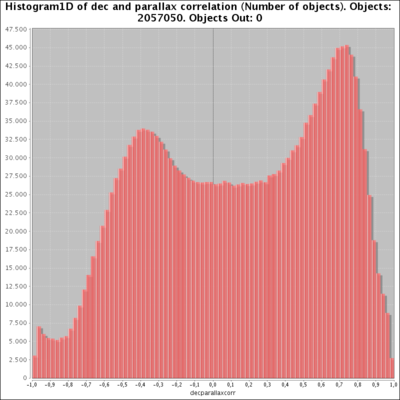Figure 7.31: Histogram of correlation between δ and ϖ (larger), between δ and μα⁣* (larger), between δ and μδ (larger), and between ϖ and μα⁣* (larger).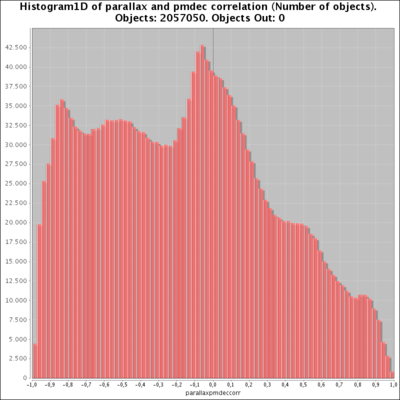Figure 7.32: Histogram of correlation between ϖ and μδ (larger), Histogram of correlation between μα⁣* and μδ (larger).Figure 7.33: HEALPix map in ecliptic coordinates of correlation between α and δ: mean value (larger), and standard deviation (larger); correlation between α and ϖ: mean value (larger), and standard deviation (larger).Figure 7.34: HEALPix map in ecliptic coordinates of correlation between α and μα⁣*: mean value (larger), and standard deviation (larger); correlation between α and μδ: mean value (larger), and standard deviation (larger).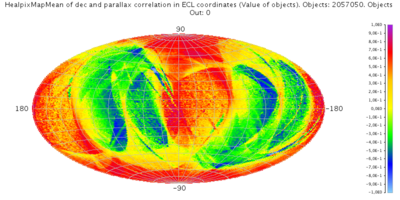Figure 7.35: HEALPix map in ecliptic coordinates of correlation between δ and ϖ: mean value (larger), and standard deviation (larger); correlation between δ and μα⁣*: mean value (larger), and standard deviation (larger).Figure 7.36: HEALPix map in ecliptic coordinates of correlation between δ and μδ: mean value (larger), and standard deviation (larger); correlation between ϖ and μα⁣*: mean value (larger), and standard deviation (larger).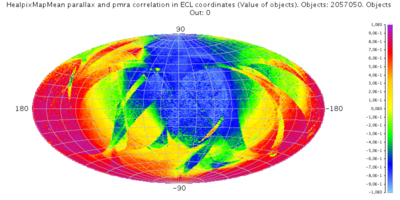Figure 7.37: HEALPix map in ecliptic coordinates of correlation between ϖ and μδ: mean value (larger), and standard deviation (larger); correlation between μα⁣* and μδ: mean value (larger), and standard deviation (larger).